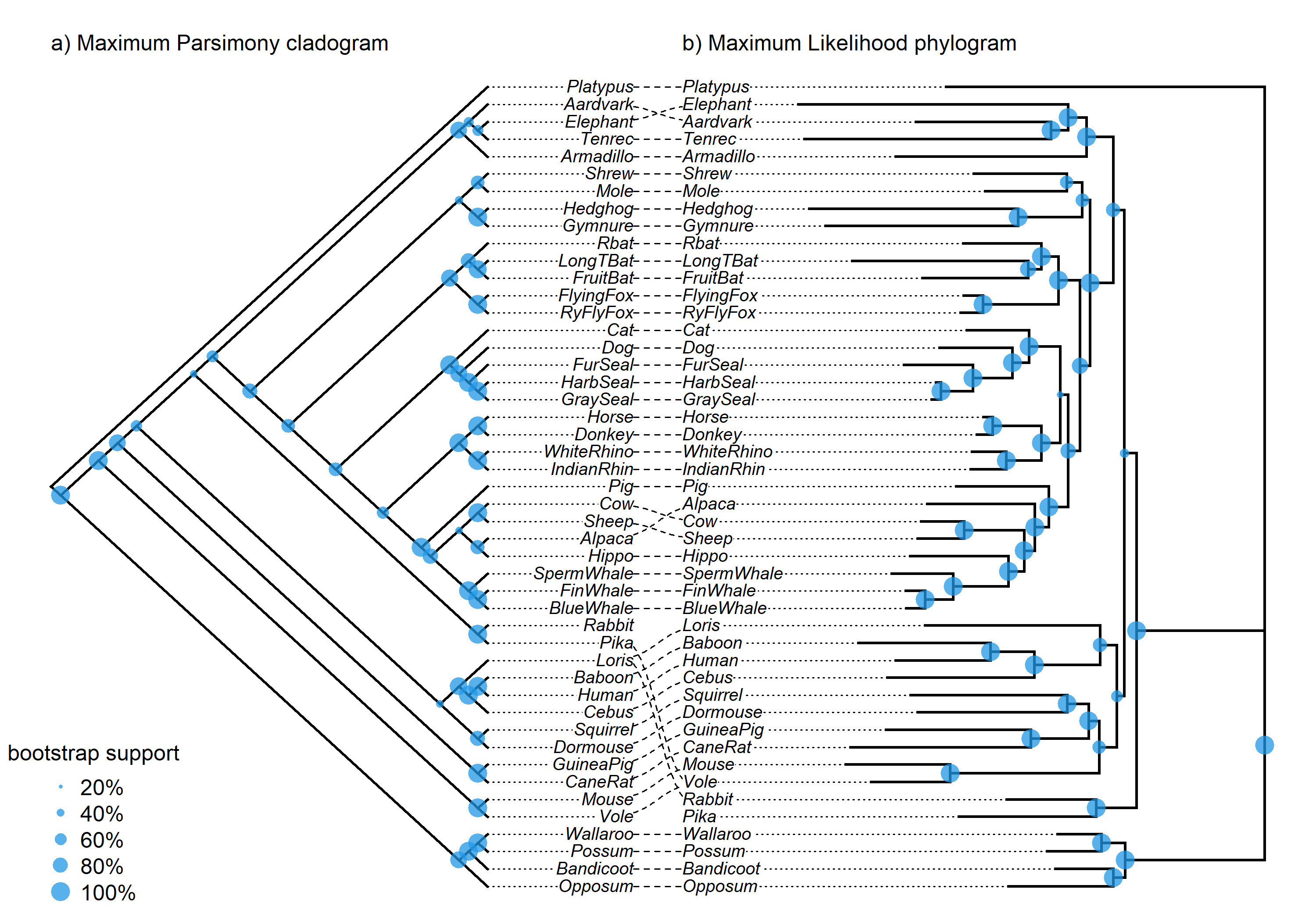## Thursday, May 11, 2023

### Follow-up to plotting node labels on a co-phylogenetic tanglegram

Yesterday I wrote a post illustrating how to preserve node labels (such as bootstrap values) on a plotted `"cophylo"` object.

I meant to include a tutorial illustrating how to plot those bootstrap proportions as differently sized points: larger points for higher bootstrap support of each clade. Here’s how.

``````library(phytools)
``````

Starting from the rooted MP & ML trees we obtained yesterday

``````rooted.mp_tree
``````
``````##
## Phylogenetic tree with 47 tips and 46 internal nodes.
##
## Tip labels:
##   Platypus, Wallaroo, Possum, Bandicoot, Opposum, Rabbit, ...
## Node labels:
##   Root, 1, 1, 0.89, 0.38, 0.39, ...
##
## Rooted; no branch lengths.
``````
``````rooted.ml_tree
``````
``````##
## Phylogenetic tree with 47 tips and 46 internal nodes.
##
## Tip labels:
##   Platypus, Wallaroo, Possum, Bandicoot, Opposum, Rabbit, ...
## Node labels:
##   Root, 1, 1, 0.722, 0.986, 0.999, ...
##
## Rooted; includes branch lengths.
``````

Let’s create our `"cophylo"` object as follows:

``````obj<-cophylo(rooted.mp_tree,rooted.ml_tree)
``````
``````## Rotating nodes to optimize matching...
## Done.
``````

Finally, we plot it.

``````plot(obj,type=c("cladogram","phylogram"),fsize=0.8,part=0.48,
left<-get("last_plot.cophylo",envir=.PlotPhyloEnv)[]
ind<-2:Nnode(obj\$trees[])+Ntip(obj\$trees[])
points(left\$xx[ind],left\$yy[ind],
cex=2*as.numeric(obj\$trees[]\$node.label[2:Nnode(obj\$trees[])]),
pch=16,col=make.transparent(palette(),0.75))
right<-get("last_plot.cophylo",envir=.PlotPhyloEnv)[]
ind<-2:Nnode(obj\$trees[])+Ntip(obj\$trees[])
points(right\$xx[ind],right\$yy[ind],
cex=2*as.numeric(obj\$trees[]\$node.label[2:Nnode(obj\$trees[])]),
pch=16,col=make.transparent(palette(),0.75))
legend("bottomleft",
legend=paste(seq(20,100,by=20),"%",sep=""),
pt.cex=seq(0.4,2,by=0.4),
pch=16,col=make.transparent(palette(),0.75),
title="bootstrap support",bty="n")